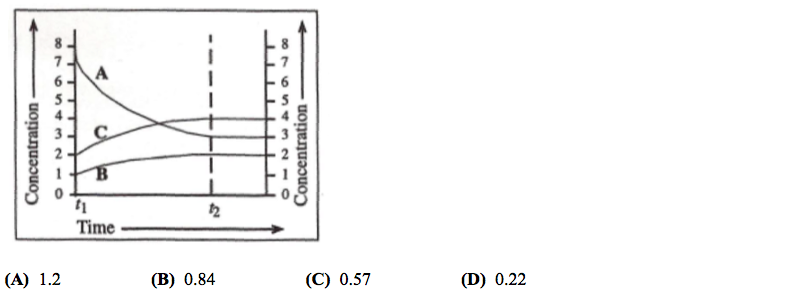# Problem: The graph shows the varation of concentration with time for this reaction at 25°C3A(aq) ⇌ B(aq) + 2C(aq)What is the value of the equilibrium constant at  t2?

###### FREE Expert Solution
90% (152 ratings)
###### Problem Details

The graph shows the varation of concentration with time for this reaction at 25°C

3A(aq) ⇌ B(aq) + 2C(aq)

What is the value of the equilibrium constant at  t2?What scientific concept do you need to know in order to solve this problem?

Our tutors have indicated that to solve this problem you will need to apply the Equilibrium Expressions concept. If you need more Equilibrium Expressions practice, you can also practice Equilibrium Expressions practice problems.

What is the difficulty of this problem?

Our tutors rated the difficulty ofThe graph shows the varation of concentration with time for ...as low difficulty.

How long does this problem take to solve?

Our expert Chemistry tutor, Jules took 1 minute and 21 seconds to solve this problem. You can follow their steps in the video explanation above.

What professor is this problem relevant for?

Based on our data, we think this problem is relevant for Professor Nishida's class at WSU.